# Spoken Digit Commands

A dataset consisting of recordings of spoken digits

The dataset contains 10,000 training and 1,000 test recordings of 10 classes corresponding to spoken digits from 0 to 9. The total number of speakers is 997. The dataset is a subset of the Speech Commands Dataset v0.01 released by Google. The selection has been done so that speakers in the training and test sets do not overlap.

## Examples

### Basic Examples

Retrieve the resource:

 In:=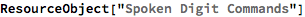Out=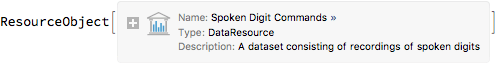Retrieve a sample of the training dataset:

 In:=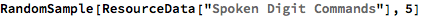Out=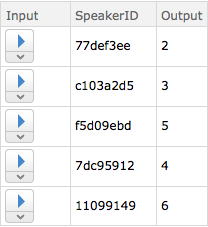Retrieve a sample of the test dataset:

 In:=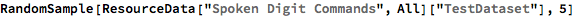Out=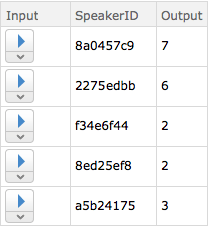### Statistics

Compute the number of examples per class:

 In:=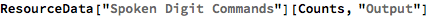Out=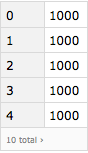Compute the total number of different speakers in the training set:

 In:=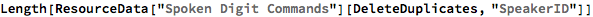Out=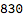Inspect the sample rate and channel count of the Audio objects:

 In:=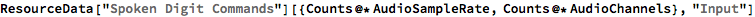Out=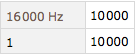Plot the histogram of the durations of the Audio objects:

 In:=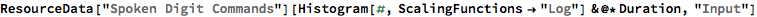Out=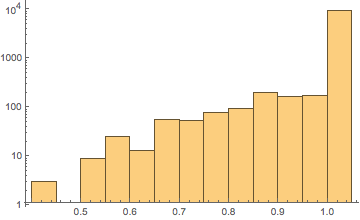### Visualization

Select an Audio object from the dataset:

 In:=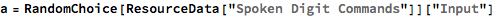Out=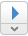Visualize the waveform:

 In:=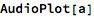Out=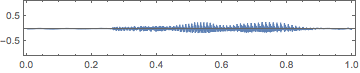Visualize the spectrum:

 In:=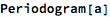Out=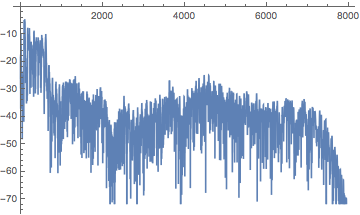Visualize the spectrogram:

 In:=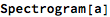Out=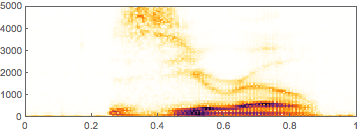Wolfram Research, "Spoken Digit Commands" from the Wolfram Data Repository (2018)